Technical Article

# Writing PICAXE BASIC Code - Part 4

September 16, 2015 by Charles R. Hampton

## Part 4 introduces the readadc, select, case, and endselect PICAXE BASIC commands. It is the fourth article in a multi-part series on writing PICAXE code.

Beginner

#### Prerequisites

Writing PICAXE BASIC Code - Part 1

Writing PICAXE BASIC Code - Part 2

Writing PICAXE BASIC Code - Part 3

This is the fourth article in a multi-part series on writing PICAXE BASIC code.

• Part 1 introduced the high, low, pause, and goto commands, the #picaxe directive, and the concept of labels.

• Part 2 introduced the for...next, wait, and symbol commands, general purpose variables, and the #no_data directive

• Part 3 introduced the if...then, endif, gosub, and return commands.

Prior to continuing with this article, completion of part 1 of this series is required. Part 1 provided complete details for the construction of the PA-08M2 Coding Test Circuit, which is essential to completion of this article. The schematic diagram is shown below for reference purposes.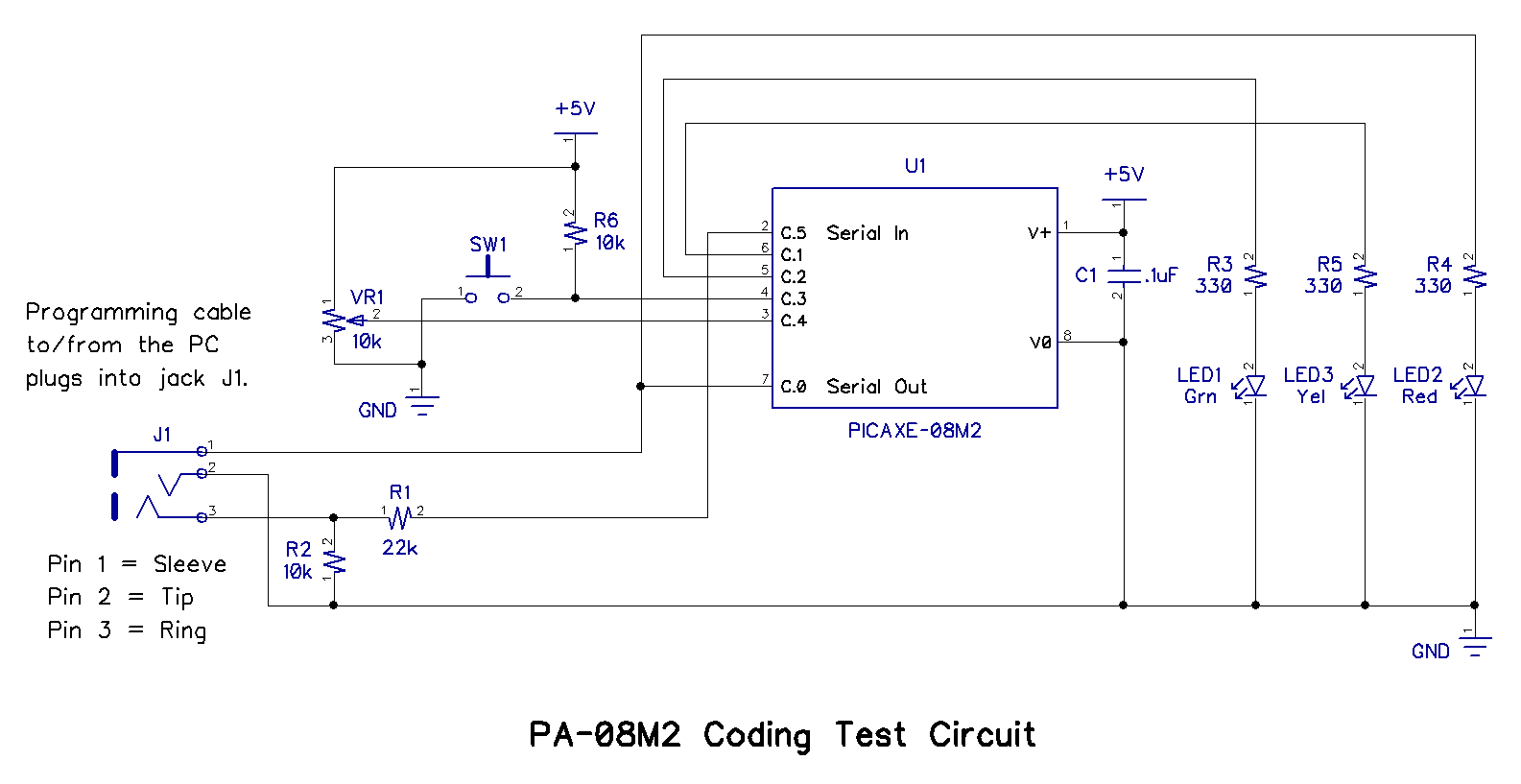### Voltage Dividers

In the PA-08M2 Coding Test Circuit, VR1 is a potentiometer (pot, for short) arranged in a voltage divider configuration. To understand its function in the circuit, it is first necessary to understand what a voltage divider is in general.

A voltage divider is a circuit that produces an output voltage that is some fraction of its input voltage. Two simple schematic examples of a voltage dividers are shown below.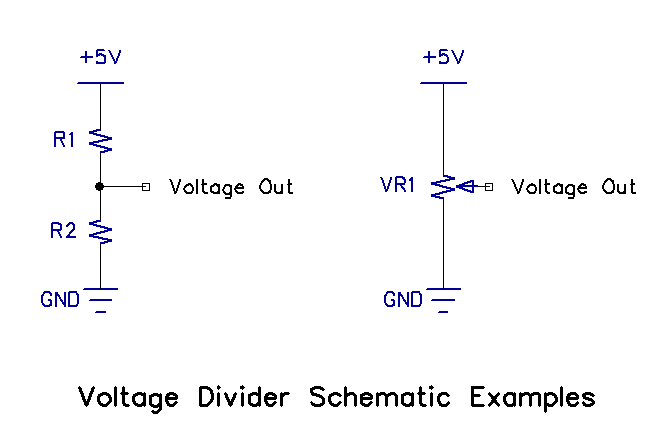The example on the left consists of two resistors connected in series with the input voltage applied across the resistor pair and the output voltage appearing at the connection between them. The output voltage will be some fraction of the input voltage and is determined by the resistance ratio of R1 and R2.

The example on the right is a potentiometer set up as a voltage divider. A pot consists of a three-terminal resistor with a sliding or rotating contact. The output voltage will be some fraction of the input voltage and is determined by the position of the sliding or rotating contact. The formula for determining the voltage out of a purely resistive voltage divider is shown following:

Vout = (R1/(R1 + R2)) x Vin

As VR1 is rotated, the voltage out changes, but it never rises above +5V (or whatever the actual supply voltage is in your circuit), and never falls below ground. Assuming the pot is linear, its output rises and falls proportionately to the position of the rotating contact, also called the "wiper." Whatever the voltage out is, that voltage is present at pinC.4 of the PICAXE 08M2.

The next logical question is what the microcontroller will do with that analog voltage. For the answer, first look at the 08M2+ pinout drawing reproduced below.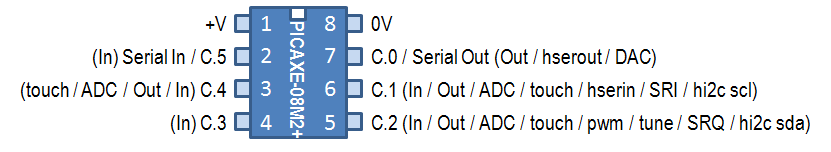PinC.4 is located on leg 3 of the PICAXE 08M2+, and as shown, pinC.4 can function as a touch input, an ADC input, a general purpose digital output, or a general purpose digital input. The capability that is needed is the ADC function; ADC is an abbreviation for Analog to Digital Converter. The 08M2 is a microcontroller, and is essentially a digital device, so the analog voltage value that is present at pinC.4 must be converted to a digital format, and that is exactly what the ADC function does.

"But," you might ask, "How does the 08M2 know that pinC.4 is supposed to be an ADC input?" Of course, it's the program code that "tells" the microcontroller what to do. Here is the code for you to download.

Potentiometer_to_LED_Speed.zip

### Program Aanlysis

The first 23 lines of the program are shown below. Lines 1 through 10 have been previously explained and need no further elucidation. Likewise lines 17 through 19 are symbol definitions, with which you should be very familiar.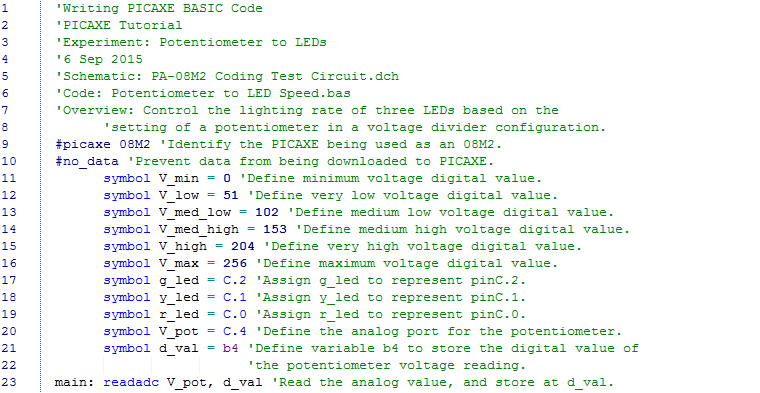Look at line 20; note that it is a symbol definition for pinC.4 of the 08M2, which is the very pin in question. PinC.4 is now referred to as "V_pot" and you see from the line 20 comment that it does indeed represent the voltage reading from potentiometer VR1 in the schematic.

As you should recall, any time data is read, a storage location must be assigned for it. That is the purpose of line 21; the voltage read from the output of VR1 will be called "d_val" and will be stored in location b4, a general purpose byte variable.

Of course, there's a problem there. The voltage reading is analog, and it must be converted to a digital format in order to be stored. Line 23 is the answer; the readadc command tells the µC to read the analog value at V_pot (which is pinC.4), convert it to digital format, and store it at d_val (which is general purpose byte variable b4). This works well because readadc creates an 8-bit digital representation of the voltage, and b4 is a byte (8-bit) variable.

An 8-bit binary number can convey 28, or 256 different data combinations, thus d_val can be anywhere from 0 (zero) to 255, depending upon the voltage level at pinC.4. That helps to explain lines 11 through 16 in the code, which assign symbols to six different values of d_val: 0, 51, 102, 153, 205, and 256. These six values effectively divide the entire range into five equal segments, as indicated by the symbol names. Keep that in mind as you look at the next section of the code, shown below.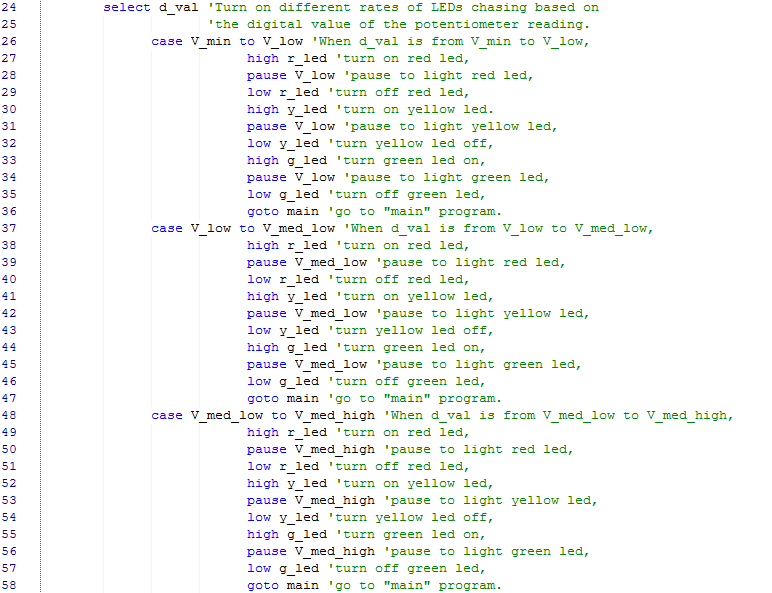Line 24 introduces the select command, and the comment in lines 24 and 25 indicates what it does. Select directs the microcontroller to compare d_val to different "cases" of what d_val could be. The first case is when d_val is at least V_min (0) but less than V_low (51), the second case is when d_val is at least V_low (51) but less than V_med_low (102), and so on to V_max.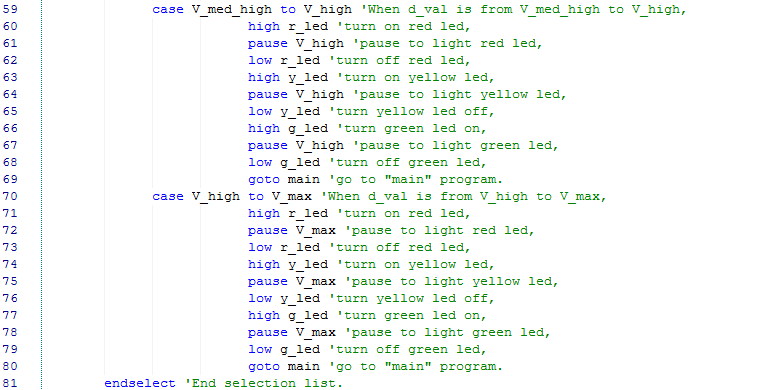Line 81 signals the PICAXE that there are no more cases to consider in response to the select d_val command in line 24.

Here's a review of the code operations thus far. The µC read the voltage at pinC.4 (V_pot), converted it to an 8-bit digital number, and stored it in general purpose byte variable b4 (d_val). It then compared d_val to the range of values defined in the first case (0 to 51), and if there was not a match, it proceeded to each case in order until a match was found.

Assume that a match was found in the last case, i.e., line 70. The PICAXE then executes the code in lines 71-78, and the LEDs turn on and off in the sequence defined and according to the pauses included.

Compare the LED lighting sequence in all five cases, and you will recognize that they are identical: red turns on, pauses, and then turns off, yellow turns on, pauses, and then turns off, finally green turns on, pauses, and then turns off. Only the pause lengths are different. For example, in the first case, the pause is defined by the symbol V_low, which is 51, and because the pause command is always denominated in milliseconds, the pause length is 51 milliseconds. The pause length in each of the five cases is defined in the same way, which results in five different pause lengths: 51 ms, 102 ms, 153 ms, 204 ms, and 255 ms. You may have noticed that both the pause lengths and the d_val ranges are determined by the symbol definitions in lines 11 through 16. This is not necessary, and was done by the author simply for convenience. The code could be improved by defining separate pause length symbols, which would allow the pauses to be changed without affecting the case ranges.

Because of the goto main command in the last line of each case sequence, the execution repeats until power is disconnected. The net result is a 3 LED "chaser," with five different chase speeds controlled by the setting of VR1. If you haven't run the code yet, you are very patient; try it now, and see for yourself.

### Things to Try

• Reverse the polarity of VR1 (by moving only two wires) and observe the effect on case selection.

• Change the code to reverse the direction in which the LEDs flash.

• Add symbol definitions to the code for the five different pause timings; then implement the new pause timings in each of the five cases.

• Make changes to the code such that instead of an LED chaser, the setting of VR1 controls which of the LEDs light as follows: lowest voltage range lights only the green LED; second highest voltage range lights the green and the yellow LEDs; middle voltage range lights the yellow LED; fourth highest voltage range lights the yellow and red LEDs; top voltage range lights the red LED. Based on the supply voltage of your circuit, calculate the voltage at which the LED combinations light. Check your results with a digital multimeter.

### Conclusion

This is the final part in Writing PICAXE BASIC Code. This series was written for beginners, and if you have completed all four parts, you are no longer a beginner. Congratulations!

Of course, that doesn't mean there isn't more to learn. PICAXEs are an amazing devices, and these articles have barely begun to explore them. Look for additional PICAXE technical articles and projects here on AAC. There's always more to come!•Charles R. Hampton February 10, 2016